Function Repository Resource:

# WelchSpectralEstimate

Fast numerical estimation of the power spectral density or the cross spectral density

Contributed by: Julien Kluge
Quantum Optical Metrology; Joint Lab Integrated Quantum Sensors
Department of Physics
Humboldt-Universität zu Berlin
julien@physik.hu-berlin.de
 ResourceFunction["WelchSpectralEstimate"][data,rate] estimates the data power spectral density at a given rate. ResourceFunction["WelchSpectralEstimate"][data1,data2,rate] estimates the data cross spectral density at a given rate.

## Details and Options

ResourceFunction["WelchSpectralEstimate"] accepts numerical data, TemporalData objects or TimeSeries objects.
ResourceFunction["WelchSpectralEstimate"] always assumes evenly spaced data points. For TimeSeries or TemporalData sets, the rate is estimated as the number of data points divided by the duration between the first and last time stamp.
Supported options are:
 "SegmentSize" Automatic the number of datapoints to use in a Fourier transform "OverlapOffset" Automatic the offset between segments to average or a fraction of the possible offset (defaults to half of the "SegmentSize") FourierParameters {0,1} Fourier convention parameters "Window" Automatic the spectral window to apply to the data (if appropriate, the HannWindow will be chosen) "OneSided" True True to evaluate only the first half of the Fourier transform, otherwise False for evaluating the whole range "Reduction" Mean the function to average the segments together "DensityScaling" True True for scaling as a density, False for spectrogram scaling "Detrend" "Constant" the level of detrending to apply to the data segments, before transformation

## Examples

### Basic Examples (1)

Calculate the spectral density of a random data sample:

 In:=Out=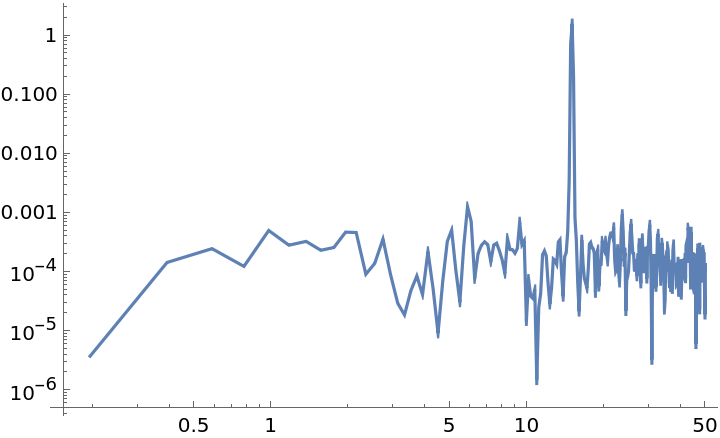### Scope (2)

Evaluate the Cross Spectral Density (CSD) of two sinusoidal, noisy datasets and display amplitude and phase:

 In:=Out=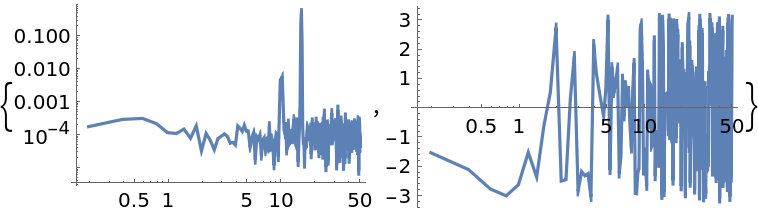Compare the influence of different spectral windows on the PSD:

 In:=Out=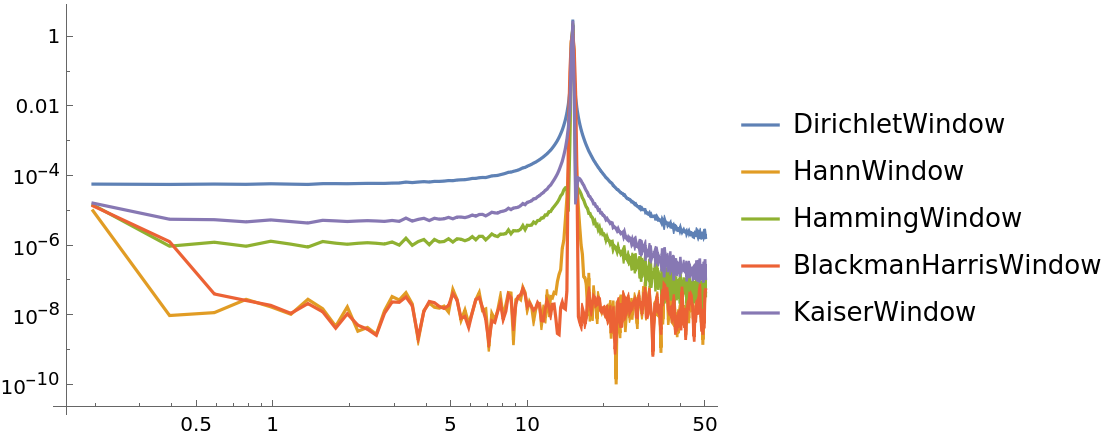### Options (12)

#### SegmentSize (1)

"SegmentSize" sets number of data points from which to calculate the Fourier transform; smaller numbers means more averaging but lower resolution:

 In:=Out=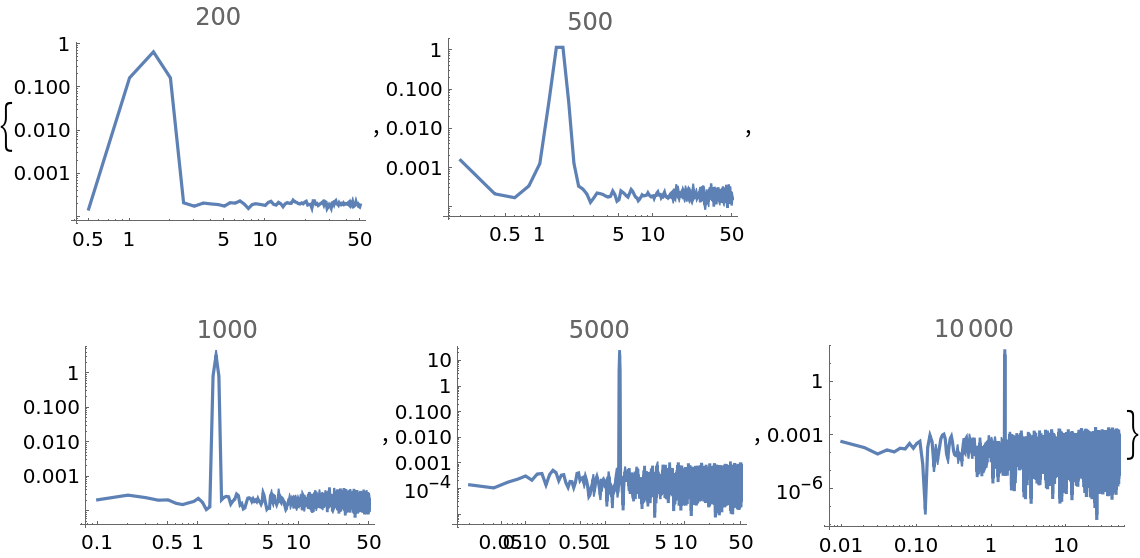#### OverlapOffset (2)

"OverlapOffset" allows to set the distance of the starting point between two partitioned segments; a smaller integer number means closer overlaps and thus more averaging:

 In:=Out=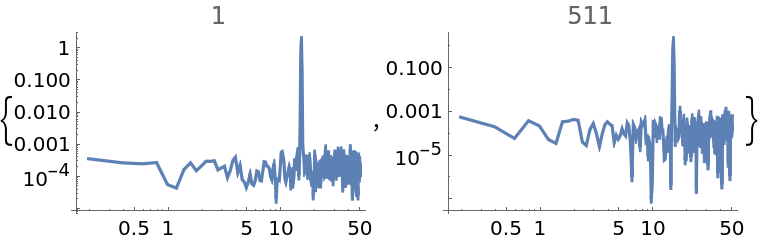"OverlapOffset" also allows the specification of an overlap fraction of the number of data points minus the segment size, as a real number between zero and one:

 In:=Out=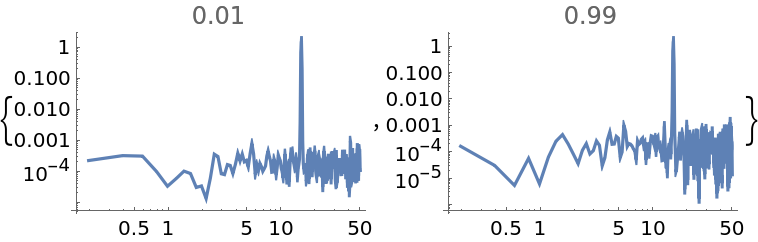#### FourierParameters (1)

Set the FourierParameters option for the respective Fourier transforms:

 In:=Out=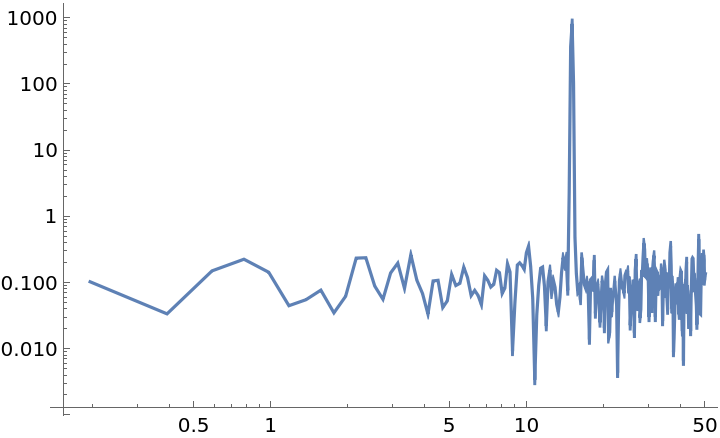#### Window (3)

Use the "Window" option to specify windowing functions:

 In:=Out=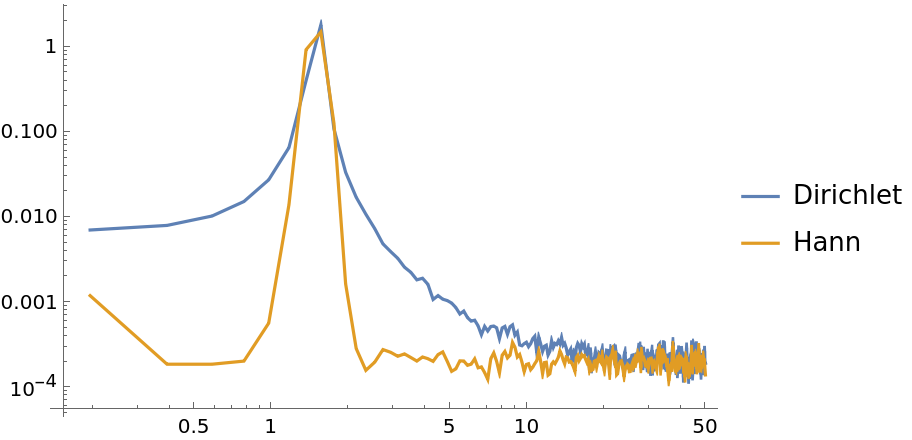A list can be applied as the window function, provided the length is equal to its segment size:

 In:=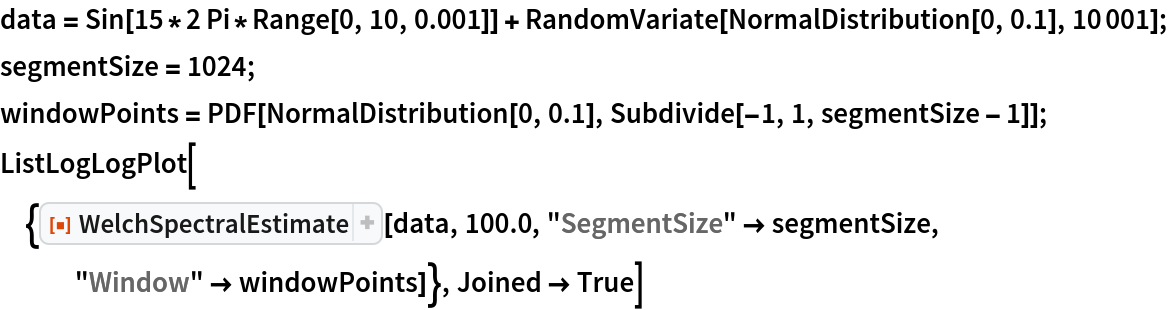Out=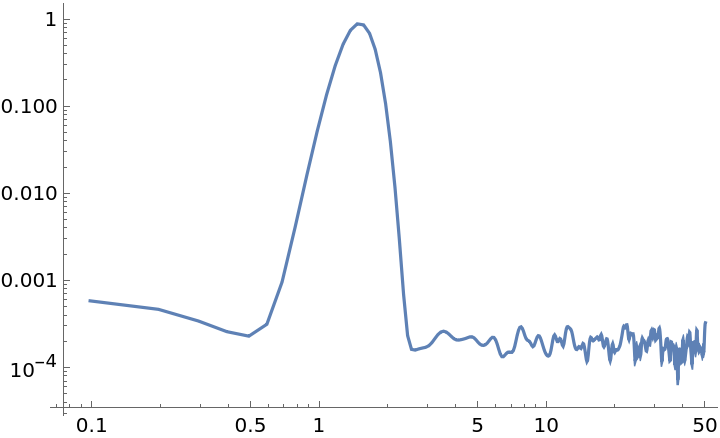Set a custom function as the window:

 In:=Out=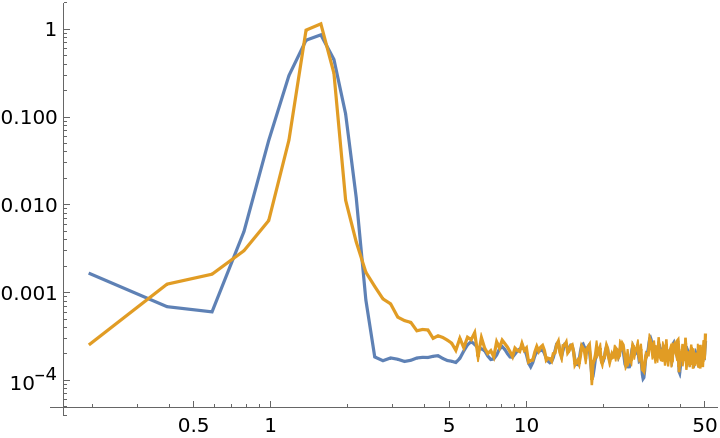#### OneSided (1)

Set "OneSided" to False to get a two sided evaluation:

 In:=Out=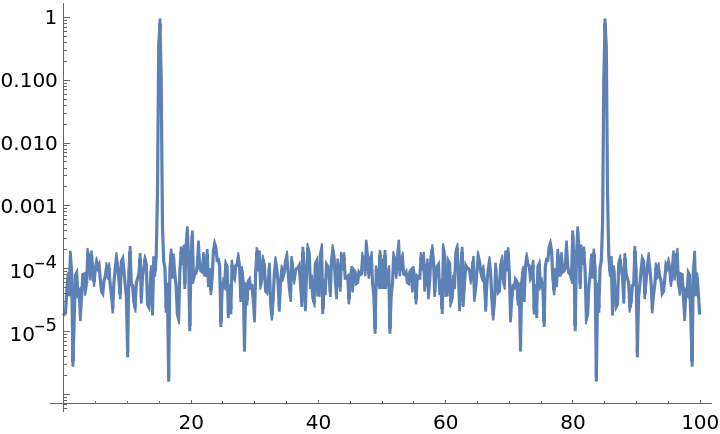#### Reduction (1)

Specify Median as a reduction function:

 In:=Out=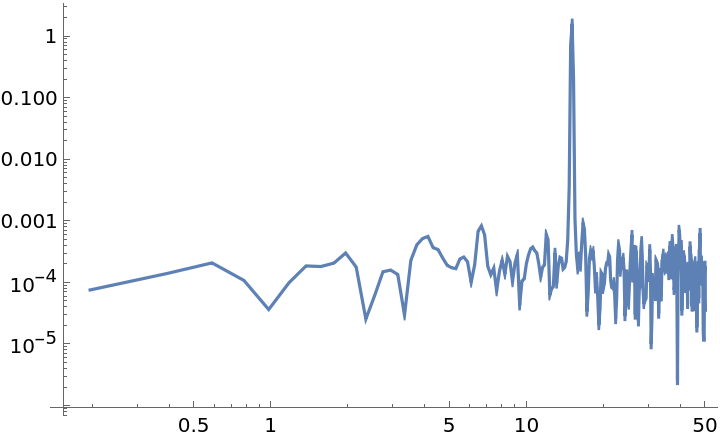#### DensityScaling (1)

Set "DensityScaling" to False to get a spectrum scaling:

 In:=Out=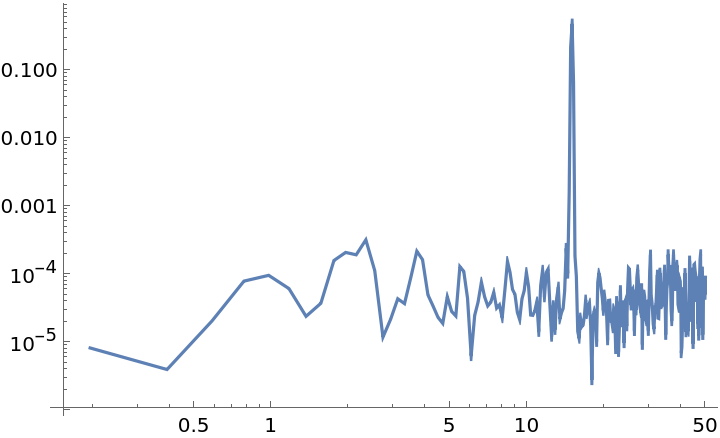#### Detrend (2)

The "Detrend" option can be used to detrend the initial data segments before transformations occur. The option can be specified with string arguments for constant or linear detrends:

 In:=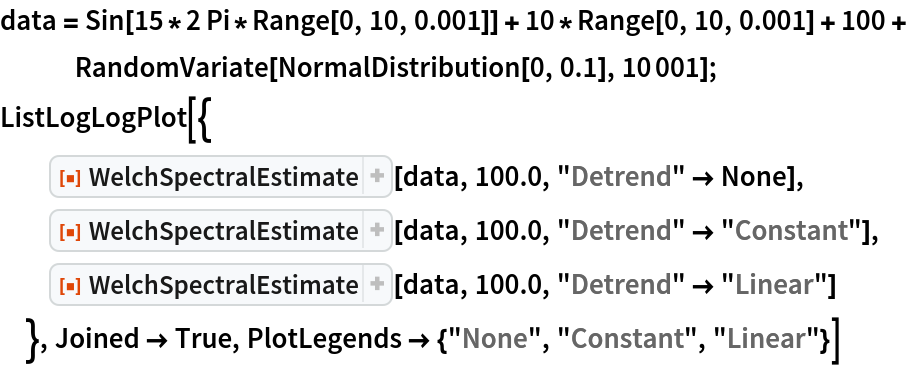Out=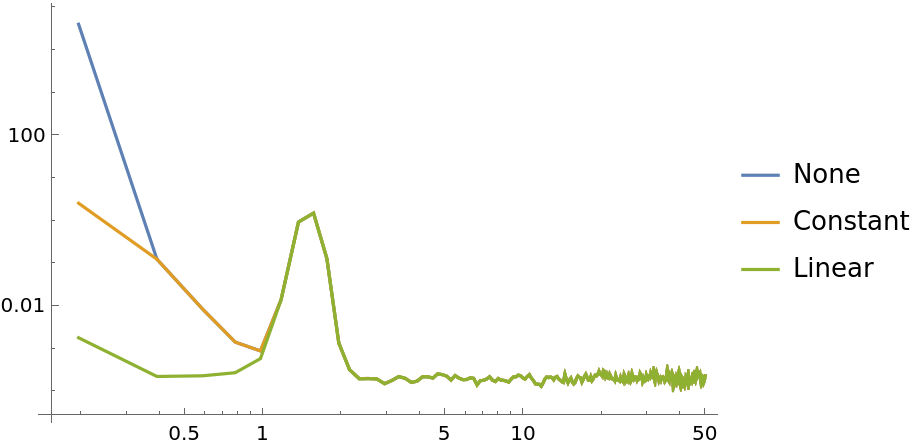A list of integers can be specified instead which polynomial orders should be detrended:

 In:=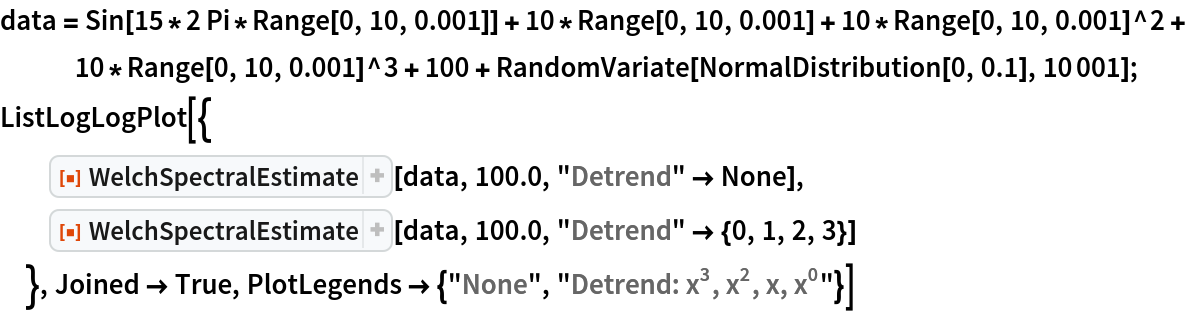Out=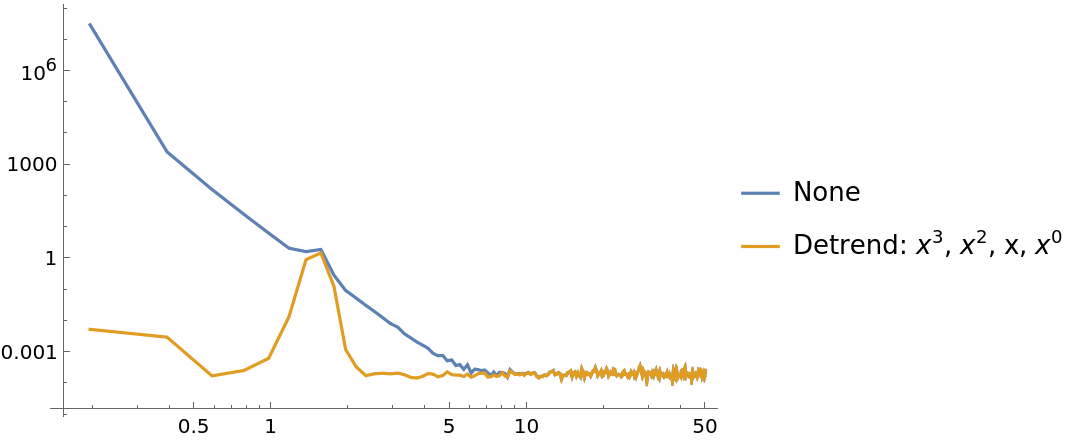### Applications (2)

Investigate the frequency response on white noise data for different kind of filters:

 In:=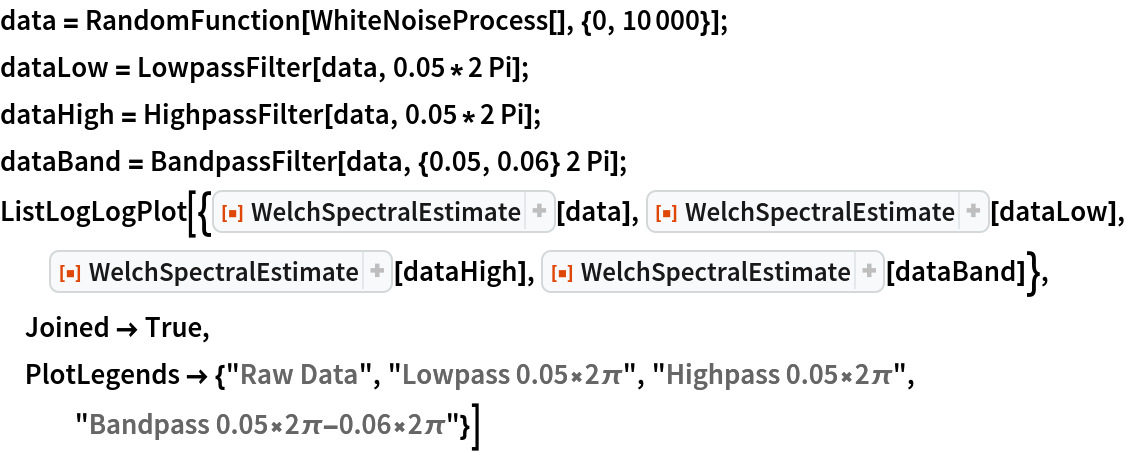Out=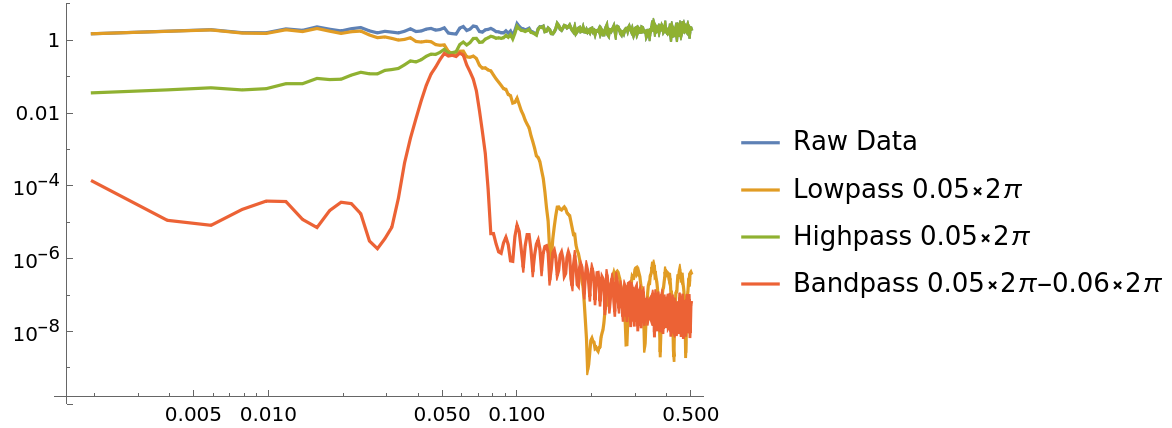Estimate the system transfer function of a set state and its measured response:

 In:=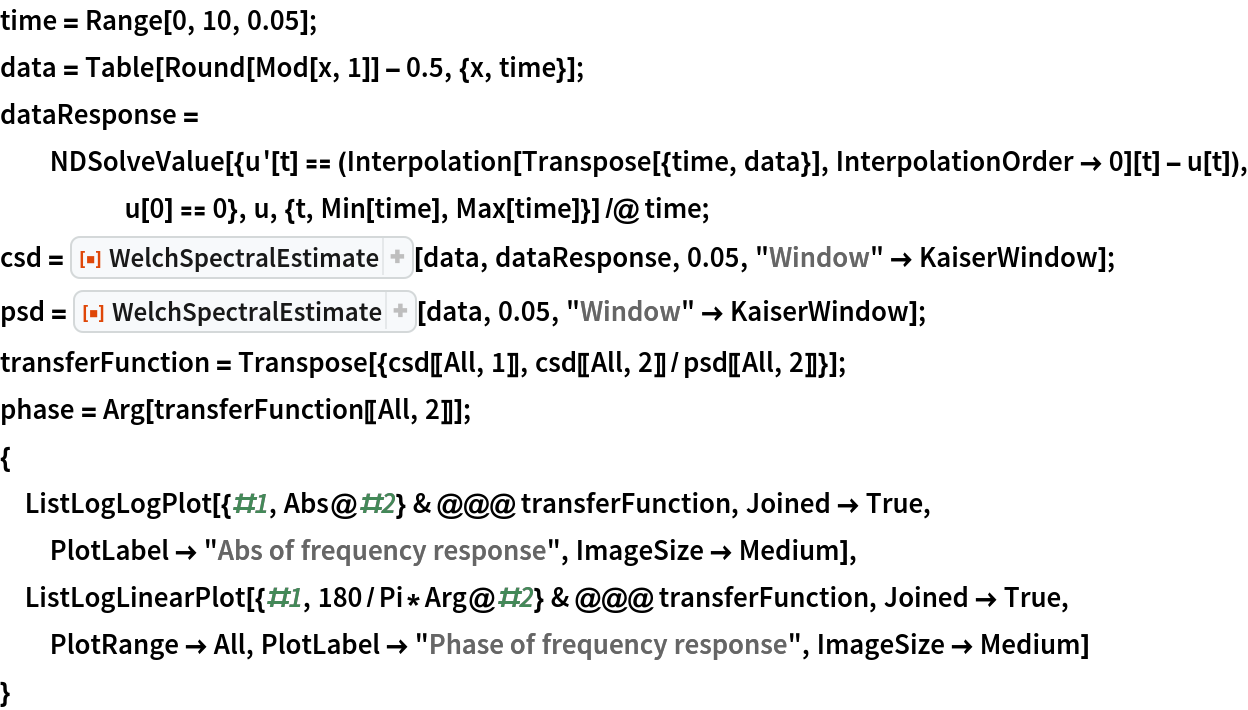Out=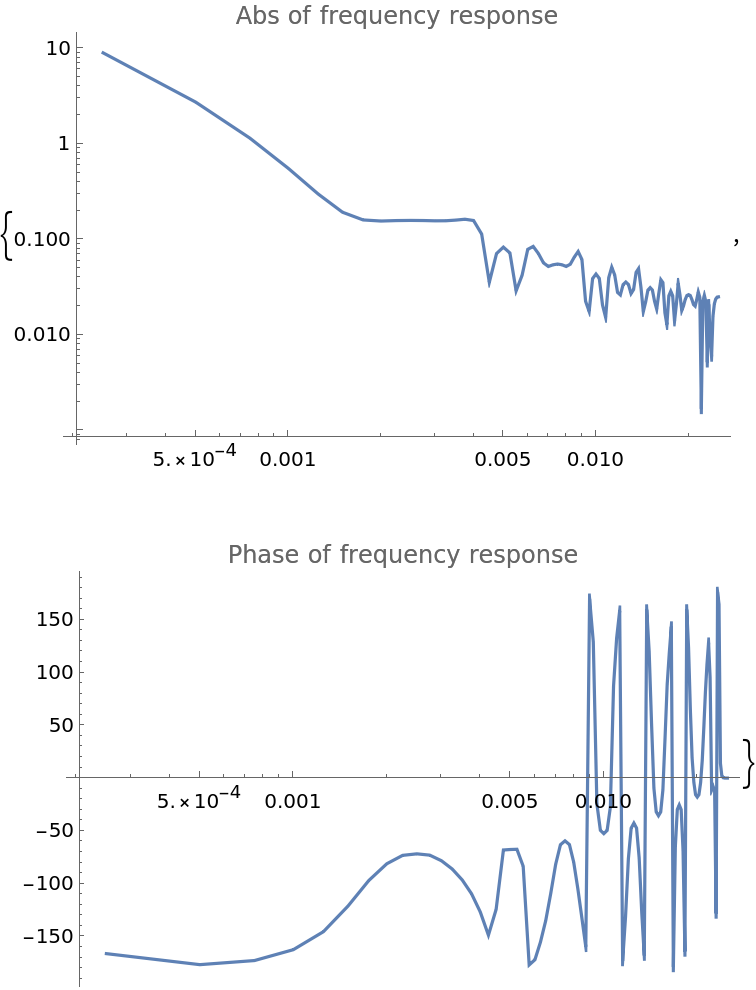### Possible Issues (1)

Even for complex input, the "OneSided" option will still remain True by default and thus return only the front of the Fourier transform:

 In:=Out=### Neat Examples (1)

Estimate the uncertainty of the Fourier deviations by just choosing a different reduction function:

 In:=Out=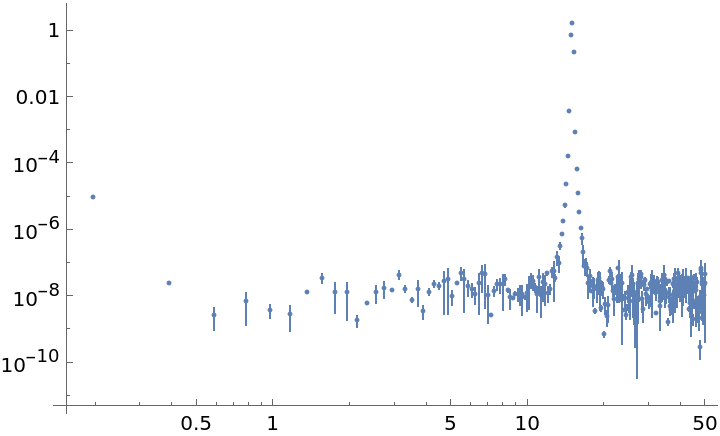Julien Kluge

## Version History

• 1.0.0 – 10 February 2021